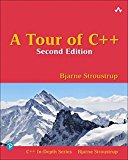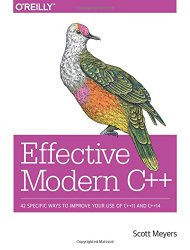# C++17 constexpr generation of a FizzBuzz solution

## Posted on September 24, 2018 by Paul

The famous FizzBuzz interview question asks to print all numbers from 1 to 100. If a number is divisible with 3 print fizz, if the number is divisible with 5 print buzz and if the number is divisible with both 3 and 5 print fizzbuzz. Writing a program for the above problem is trivial. A more interesting problem is to generate the solution for the FizzBuzz problem at compile time.

In C++ we use the constexpr specifier for a variable or a function that needs to be evaluated at compile time.

A possible approach for the FizzBuzz problem is to use a two dimensional array of characters that is filled with data during the compilation. The array will have 100 rows, one for each number from 1 to 100 and 9 columns. Here is an example of how the solution could be stored in the above array:

Let’s start by defining the dimensions of the solution array:

Next, we need a function that will convert an integer to a constexpr one-dimensional array of characters:

Please note, that the above code does not check if the provided number can fit in the available space, for our particular problem a 9 columns array is more than enough.

Now, we can store the solution in a two-dimensional constexpr array:

We can check that the solution is actually generated at compile time by using static_assert, e.g.:

If you want to print the solution, you can do it at run time, e.g.:

The above code was checked with GCC 8.2, Clang 7 and a preview of the next MSVC compiler. You can see the compilation options and the generated assembly for the 3 test compilers at https://godbolt.org/z/hTmXFS.

Here is an example of running the above code on a macOS machine: